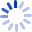An Introduction to Categorical Data Analysis

A non-technical introductory featuring the most important techniques for analyzing categorical data such as classical inferences for two-and-three way contingency tables, logistic regression, log linear models and matched-pairs data. Contains more than 200 exercises and more than 100 examples of authentic data sets. An appendix describes the use of computer packages to perform analyses in the text.

Cash for Textbooks